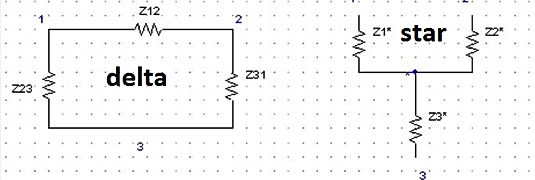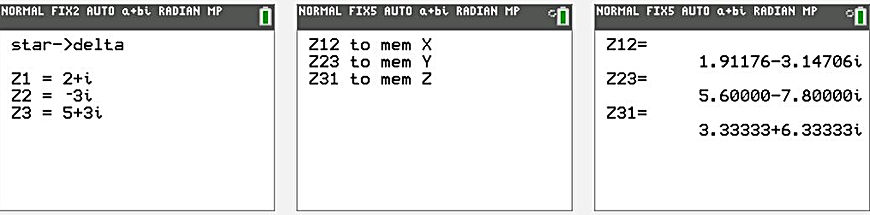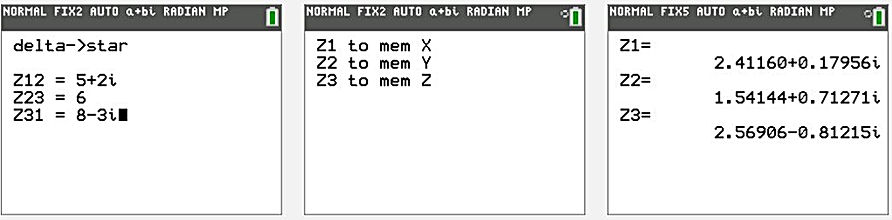naver-site-verification: naver47238a1b6bfbb19a2fd4b619734fa9a6.html
top of page

# Delta star, star-delta transformation with Ti-84 Plus (CE)

## Delta->star and star->delta conversion program of an electric network

The Delta→Star and Star→Delta conversion of an electric network require intensives calculations. This program saves a lot of time and can be used for complex networks as well as DC systems with only resistors, making it very useful in electrical engineering.

See the schematics.

Formulas :

delta→star : N=Z12+Z23+Z31,     Z1=(Z12*Z31)/N,   Z2=(Z12*Z23)/N,   Z3=(Z31*Z23)/N

star→ delta : T=Z1Z2+Z2Z3+Z3Z1,    Z12 =T/Z3,      Z23 = T/Z1,       Z31 = T/Z2

### The Electric circuits of delta and star configuration